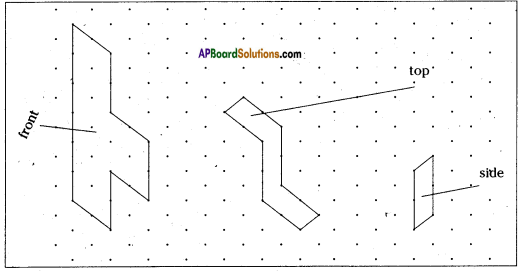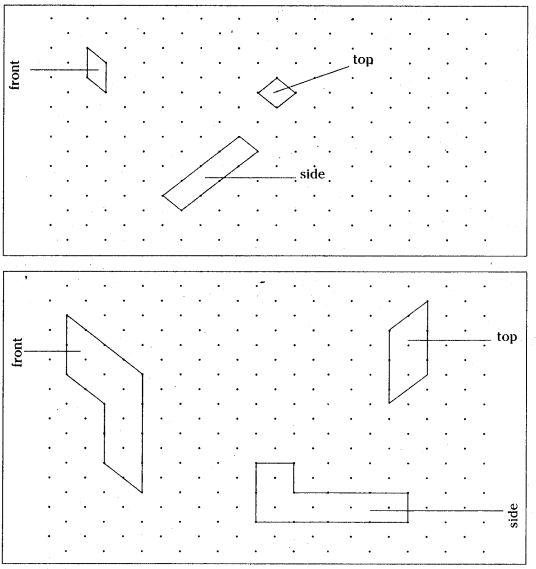AP State Syllabus AP Board 8th Class Maths Solutions Chapter 13 Visualizing 3-D in 2-D Ex 13.1 Textbook Questions and Answers.

## AP State Syllabus 8th Class Maths Solutions 13th Lesson Visualizing 3-D in 2-D Exercise 13.1Question 1.
Draw the following 3-D figures on isometric dot sheet.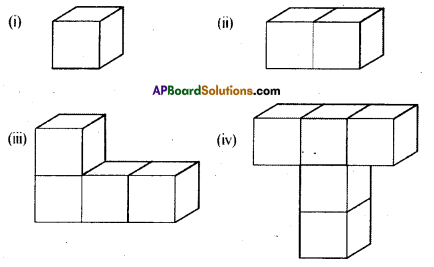Solution: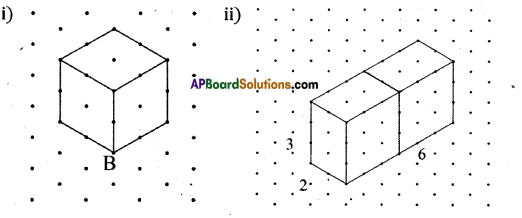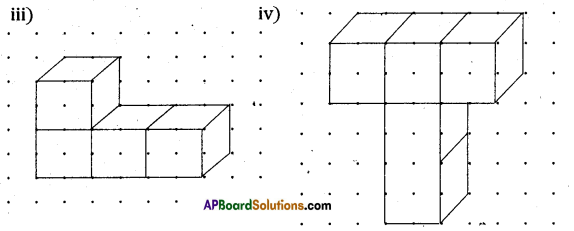Question 2.
Draw a cuboid on the isometric dot sheet with the measurements 5 units × 3 units × 2 units.
Solution: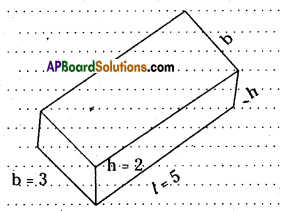Question 3.
Find the number of unit cubes in the following 3-D figures.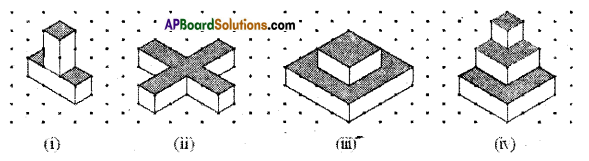Solution:

 Figure No. of cubes i) 2 + 3 = 5 ii) 2 × 4 + 1 = 9 iii) 4 + 16 = 20 iv 1 + 4 + 9 = 14Question 4.
Find the areas of the shaded regions of the 3-D figures given in question number 3.Solution:

 Figure Area of the shaped regions i) 3 × 1 × 1 =3 Sq. Units. ii) 4(2 × 1) + 1 = 9 Sq. Units. iii) 4 + (16 – 8) = 4 + 8= 12 Sq. Units. iv) 1 + (4 – 1) ÷ (9 – 4) = 1 + 3 + 5 = 9 Sq.Units.

Question 5.
Consider the distance between two consecutive dots to be 1 cm and draw the front view, side view and top view of the following 3-D figures.Solution: